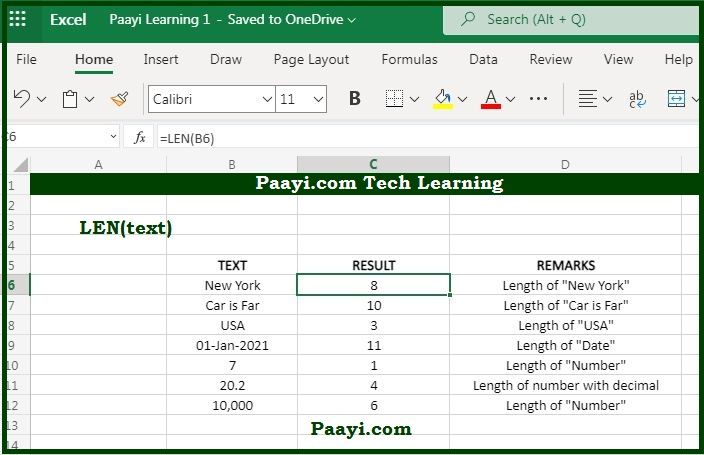# Learn How to Use Microsoft Excel LEN Function

Written by | 0 Comments | 625 Views

In this article, you will learn how to use the Microsoft Excel LEN function and its prime function in Microsoft Excel. You will also get to know the Microsoft Excel LEN function return value and syntax with the help of some examples.

## Microsoft Excel LEN Function

The main objective of the LEN function is to get the length of the text. That means with the help of the Microsoft Excel LEN function; you can able to get the return value as the length of the provided text string in the form of a number of characters. You can also be able to count the characters in the numbers with the LEN function but won't be able to include the number formatting.

### LEN Function Return Value

It will return the number of characters.

### LEN Function Syntax

= LEN(text)

Where the argument:

• text: It is the text for which you want to calculate the length.

## How to Use Microsoft Excel LEN Function?The LEN function is very useful when you want to count the number of characters in a given text. You can also be able to return the length of "numbers and dates." You won't be able to do the number formatting in the LEN function, as the length of "200"  formatted as "\$200.00" is still 3.